# COVID-19

Grid List
\$18.77
Increase value Decrease value
\$108.79
Increase value Decrease value
\$36.49
Increase value Decrease value
\$254.84
Increase value Decrease value
\$16.81
Increase value Decrease value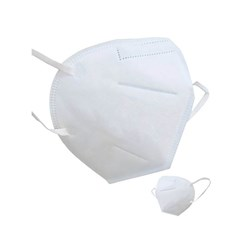\$128.70
Increase value Decrease value
\$22.00
Increase value Decrease value
\$132.00
Increase value Decrease value
\$194.70
Increase value Decrease value
\$45.87
Increase value Decrease value
\$10.78
Increase value Decrease value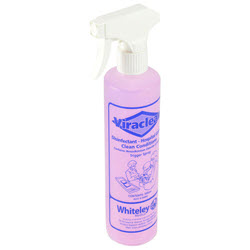\$22.63
Increase value Decrease value
\$81.36
Increase value Decrease value
\$8.71
Increase value Decrease value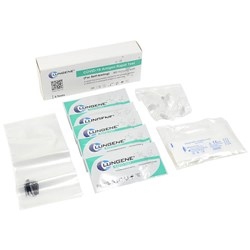From
Increase value Decrease value
\$4.95
Increase value Decrease value
\$59.00
Increase value Decrease value
Alco Wipes (200)
In Stock
\$10.62
Increase value Decrease value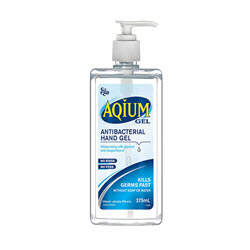\$11.68
Increase value Decrease value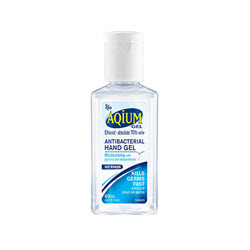\$5.68
Increase value Decrease value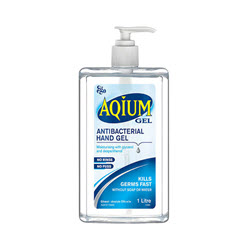\$24.24
Increase value Decrease value
\$39.60
Increase value Decrease value
\$46.20
Increase value Decrease value
\$110.75
Increase value Decrease value
\$114.00
Increase value Decrease value
\$39.23
Increase value Decrease value
\$13.68
Increase value Decrease value
\$44.25
Increase value Decrease value
\$24.78
Increase value Decrease value
\$19.75
Increase value Decrease value
\$17.03
Increase value Decrease value
\$199.65
Increase value Decrease value
\$217.80
Increase value Decrease value
\$180.86
Increase value Decrease value
\$21.74
Increase value Decrease value
\$17.29
Increase value Decrease value
From
Increase value Decrease value
From
Increase value Decrease value
From
Increase value Decrease value
From
Increase value Decrease value
Grid List
##### Products to compare:
Comparing Products